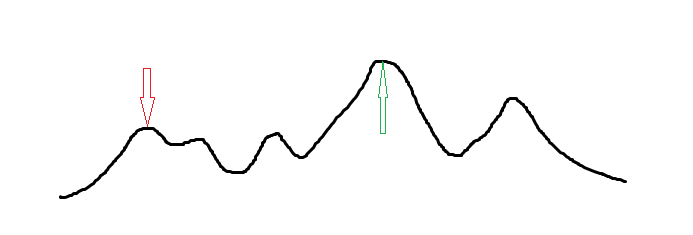# 爬山算法

## 简介

Q：你都知道是单峰函数了为什么不三分呢

A：在多年的 OI 生活中，我意识到了，人类是有极限的，无论多么工于心计，绞尽脑汁，状态总是表示不出来的，出题人的想法总是猜不透的，边界总是写不对的——所以——我不三分了 JOJO！## 具体实现

### 例 1 「JSOI2008」球形空间产生器

1. 初始化球心为各个给定点的重心（即其各维坐标均为所有给定点对应维度坐标的平均值），以减少枚举量。
2. 对于当前的球心，求出每个已知点到这个球心欧氏距离的平均值。
3. 遍历所有已知点。记录一个改变值 （分开每一维度记录）对于每一个点的欧氏距离，如果大于平均值，就把改变值加上差值，否则减去。实际上并不用判断这个大小问题，只要不考虑绝对值，直接用坐标计算即可。这个过程可以形象地转化成一个新的球心，在空间里推来推去，碰到太远的点就往点的方向拉一点，碰到太近的点就往点的反方向推一点。
4. 将我们记录的 乘上温度，更新球心，回到步骤 2
5. 在温度小于某个给定阈值的时候结束。

  1 2 3 4 5 6 7 8 9 10 11 12 13 14 15 16 17 18 19 20 21 22 23 24 25 26 27 28 29 30 31 32 33 34 35 36 #include using namespace std; double ans, cans, dis, tot, f; int n; void check() { tot = 0; for (int i = 1; i <= n + 1; i++) { dis[i] = 0; cans[i] = 0; for (int j = 1; j <= n; j++) dis[i] += (f[i][j] - ans[j]) * (f[i][j] - ans[j]); dis[i] = sqrt(dis[i]); // 欧氏距离 tot += dis[i]; } tot /= (n + 1); // 平均 for (int i = 1; i <= n + 1; i++) for (int j = 1; j <= n; j++) cans[j] += (dis[i] - tot) * (f[i][j] - ans[j]) / tot; // 对于每个维度把修改值更新掉，欧氏距离差*差值贡献 } int main() { cin >> n; for (int i = 1; i <= n + 1; i++) for (int j = 1; j <= n; j++) { cin >> f[i][j]; ans[j] += f[i][j]; } for (int i = 1; i <= n; i++) ans[i] /= (n + 1); // 初始化 for (double t = 10001; t >= 0.0001; t *= 0.99995) { // 不断降温 check(); for (int i = 1; i <= n; i++) ans[i] += cans[i] * t; // 修改 } for (int i = 1; i <= n; i++) printf("%.3f ", ans[i]); } 

### 例 2 「BZOJ 3680」吊打 XXX

  1 2 3 4 5 6 7 8 9 10 11 12 13 14 15 16 17 18 19 20 21 22 23 24 25 26 27 28 29 30 31 32 33 34 35 #include #include const int N = 10005; int n, x[N], y[N], w[N]; double ansx, ansy; void hillclimb() { double t = 1000; while (t > 1e-8) { double nowx = 0, nowy = 0; for (int i = 1; i <= n; ++i) { double dx = x[i] - ansx, dy = y[i] - ansy; double dis = sqrt(dx * dx + dy * dy); nowx += (x[i] - ansx) * w[i] / dis; nowy += (y[i] - ansy) * w[i] / dis; } ansx += nowx * t, ansy += nowy * t; if (t > 0.5) t *= 0.5; else t *= 0.97; } } int main() { scanf("%d", &n); for (int i = 1; i <= n; ++i) { scanf("%d%d%d", &x[i], &y[i], &w[i]); ansx += x[i], ansy += y[i]; } ansx /= n, ansy /= n; hillclimb(); printf("%.3lf %.3lf\n", ansx, ansy); return 0; }